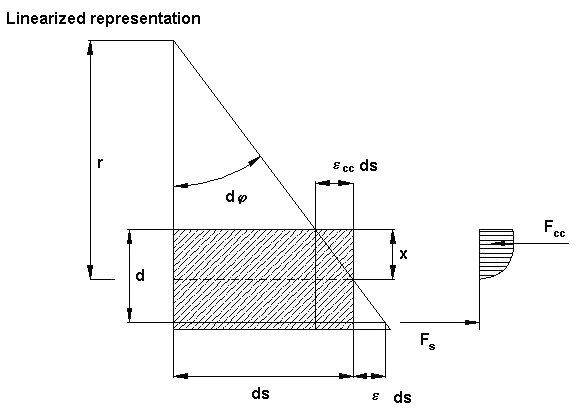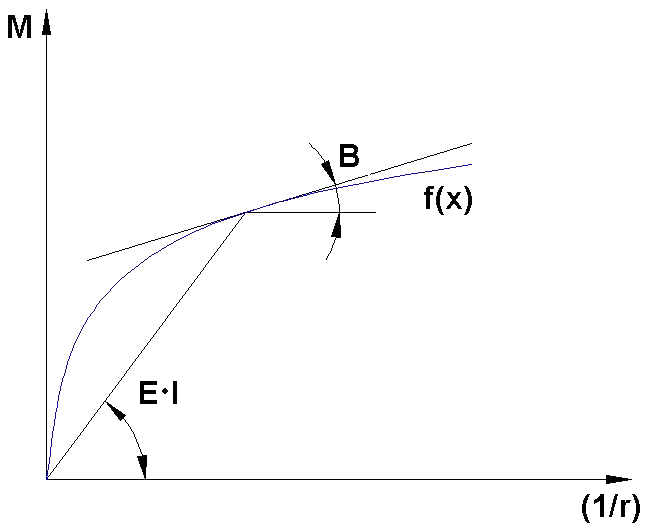# RF-CONCRETE Members – Online Manual Version 5

Online manuals, introductory examples, tutorials, and other documentation.

# 2.4.2 Strain and Curvature

### Strain and Curvature

This chapter describes the determination of significant parameters on the cross-section level. The description is reduced to a simple rectangular cross-section affected by uniaxial bending. The advantage is that the moment-curvature (axial force) relation, which mirrors the stiffness development depending on the loading the most clearly, is specified completely. The moment-curvature diagram is therefore dependent on the cross-section's loading due to axial force.

Chapter 2.4.7.1 and Chapter 2.4.8 describe the material properties that are applied for the ultimate and serviceability limit states in detail.

The following essential relations exist between strain and curvature:Figure 2.19 Relation between strain and curvature on infinitesimal element

The following conditions result based on the relations shown above.

The following results by equating

where

• εcc : negative for compressive strain of concrete

Taking the linear elastic material behavior as a basis, the relation between moment and curvature for uncracked sections (state I) is as follows.

For cracked sections (state II), the direct affinity between the course of the moment graph and curvature graph is lost. The value E ⋅ I (flexural resistance of secant) depends on the loading and is thus no longer constant where identical geometric boundary conditions are given.

The following figure illustrates the basic difference between secant and tangent stiffness.Figure 2.20 Secant stiffness (E ⋅ I) and tangent flexural stiffness (B)

When calculating deformations, the approach depends strongly on the used method. In , Quast points out the advantages of using the transfer matrix method applying the approach of the tangential flexural resistances (for area-by-area linearization (1/r)0 + M/BII). This may be very practical in regards to the mentioned method or for "manual calculations" when deformations or release rotations are to be determined with the principle of virtual work.

When the finite element method is used, calculation based on constant equivalent stiffnesses is recommended. In order to also determine the nonlinear diagram of the cross-section's moment-curvature relation in the area where abrupt changes of the tangential flexural stiffness occur with a sufficient level of detail, a finer division in such transition zones (Mcr; My) is required. This is done in the program's background by limiting the differences in stiffness of adjacent elements.

Literature
  Quast, Ulrich. Zum nichtlinearen Berechnen im Stahlbeton- und Spannbetonbau. Beton und Stahlbetonbau, Heft 9 und Heft 10, 1994.